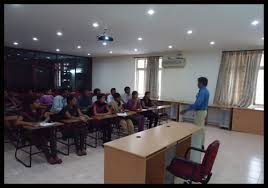Analog-Digital Hybrid Modulation

Published on Sep 29, 2021

Abstract

This paper seeks to present ways to eliminate the inherent quantization noise component in digital communications, instead of conventionally making it minimal. It deals with a new concept of signaling called the Signal Code Modulation (SCM) Technique. The primary analog signal is represented by: a sample which is quantized and encoded digitally, and an analog component, which is a function of the quantization component of the digital sample.

The advantages of such a system are two sided offering advantages of both analog and digital signaling. The presence of the analog residual allows for the system performance to improve when excess channel SNR is available. The digital component provides increased SNR and makes it possible for coding to be employed to achieve near error-free transmission.

Introduction

Let us consider the transmission of an analog signal over a band-limited channel. This could be possible by two conventional techniques: analog transmission, and digital transmission, of which the latter uses sampling and quantization principles. Analog Modulation techniques such as Frequency and Phase Modulations provide significant noise immunity as known and provide SNR improvement proportional to the square root of modulation index, and are thus able to trade off bandwidth for SNR.

The SCM Technique : An Analytical Approach

Suppose we are given a bandlimited signal of bandwidth B Hz, which needs to be transmitted over a channel of bandwidth Bc with Gaussian noise of spectral density N0 watts per Hz. Let the transmitter have an average power of P watts. We consider that the signal is sampled at the Nyquist rate of 2B samples per second, to produce a sampled signal x(n).

Next, let the signal be quantized to produce a discrete amplitude signal of M=2b levels. Where b is the no. of bits per sample of the digital symbol D, which is to be encoded. More explicitly, let the values of the 2b levels be, q1, q2, q3, q4…qM which are distributed over the range [-1, +1], where is the proportionality factor determined relative to the signal. Given a sample x(n) we find the nearest level qi(n). Here, qi(n) is the digital symbol and xa(n)= x(n)-qi(n) is the analog representation. The exact representation of the analog signal is given by x(n)=qi(n)+xa(n).

We can accomplish the transmission of this information over the noisy channel by dividing it into two channels: one for analog information and another for digital information. The analog channel bandwidth is Ba= aB, and the digital channel bandwidth being Bd= dB, where Ba+Bd=Bc, the channel bandwidth. Let =Bc/B, be the bandwidth expansion factor, i.e. the ratio of the bandwidth of the channel to the bandwidth of the signal.

Similarly, the variables a and d are the ratios of Ba/B and Bd/B. Here we will assume that a=1 so that d= -1. The total power is also divided amongst the two channels with fraction pa for the analog channel and fraction pd for the digital one, so that pa+pd=1.

 Are you interested in this topic.Then mail to us immediately to get the full report. email :- contactv2@gmail.com